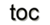Next: 2.4 Finite Differences Up: 2. Numerical Discretization Schemes Previous: 2.2 Finite Volumes

Subsections

# 2.3 Finite Elements

The finite element method is a systematic approach to approximate the unknown exact solution of a partial differential equation based on basis functions and the projection of a given domain onto a consistent finite cell complex. The finite elements correspond to the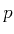-cells of the complex.

The origin of the finite element method is in the solid mechanics of rigid bodies . Some constraints should be placed on the selection of the shape functions to guarantee the fact that with an arbitrary number of shape functions the exact solution is approximated best, and in the limit, the exact solution should be obtained.

A good approximation is obtained by a residual formulation where the residuum is formulated as the difference of the unknown exact solution and the calculated approximate solution. This residuum is weighted over the simulation domain and integrated with the requirement that the integral vanishes with a set of linearly independent weighting functions. The other possible mechanism is the variational formulation of the partial differential equation [28,68].

One of the main advantages of the finite element method is the possibility to adapt the basis functions to the eigenfunctions of the differential operators . Thereby a high precision of this method can be obtained with a moderate number of mesh elements. Different areas of materials as well as anisotropic, inhomogeneous, and non-linear quantities can be treated. If no boundary conditions are declared, homogeneous/natural Neumann boundary conditions are implicitly given.

## 2.3.1 Basic Concepts for a Galerkin Method

In the following, a theoretical part of the finite element method is summarized, which is a special Galerkin method [28,29] based on the following construction of finite dimensional subspaces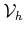:

•is triangulated into a consistent cell complex
•consists of piecewise polynomials
•has a finite basis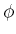with local support

The main part of this section and the corresponding notation is based on [69,29]. Galerkin's method can be briefly explained as a general technique for the construction of solution approximations not represented by infinite space basis functions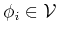but instead by a finite dimensional space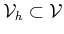of variational problems. The examples presented in this work are modeled by the following general form: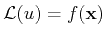(2.22)

which is defined on an arbitrary dimensional, single connected domainwith boundary. Equation 2.22 is fulfilled by functions of class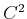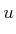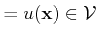(2.23)

The notation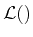being a linear spatial differential operator. Additionally, it is assumed that the domainhas a piecewise smooth boundary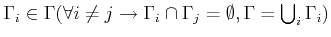. A general form of boundary conditions can thereby be specified by: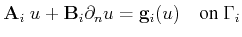(2.24)

where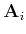and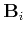are matrices consisting of functions sufficiently smooth on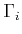and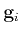is a vector of continuous linear functionals.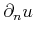denotes the outward normal derivative. A weak formulation of Equation 2.22 is obtained by using a test function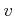: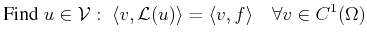(2.25)

The infinite-dimensional space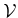is then replaced by a sequence of finite-dimensional spaceswith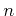linearly independent functionswhich span the space, e.g.,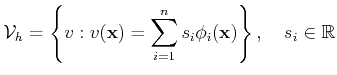(2.26)

The basis should build a complete function system to guarantee the approxicatiom for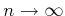. Any function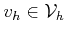is uniquely determined by a finite number of degrees of freedom, e.g., function values. This results in the following discrete variational problem for conforming methods ():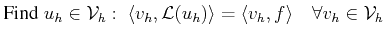(2.27)

By selecting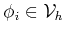the following system is obtained: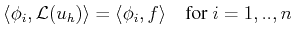(2.28)

The solution variable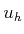is then also expanded in terms of the basis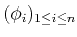of.(2.29)

A graphical representation ofis given in Figure 2.7.By the given selection of the test function and the expansion of the solution variable, it can be observed  that the scalar product in Equation 2.28 transforms the differential operatorinto the discrete operator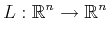(2.30)

The residuum is obtained by using the operator of Equation 2.30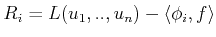(2.31)

The next step for the discrete approximation of a given problem is the subdivision of the domainaccording to cell complex properties (see Section 1.3 for details). The elements of the cell complex, the collection of cells, is then used as finite elements for the finite space. The basis functionsare defined on the global vertices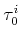, the 0-cells, only and are therefore called nodal basis functions. They can be expressed as: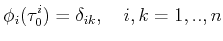(2.32)

Based on this subdivision of the finite element space, the function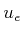can be expressed in local cell terms by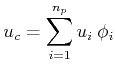(2.33)

where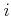represents the local index of the element and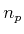the number of element vertices. Now the operator from Equation 2.30 can be defined locally for, e.g., a 3-simplex (tetrahedron):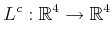(2.34)

and the local residuum is then defined as:(2.35)

To determine the operator given in Equation 2.35 for a particular cell type, the basic nodal functions have to be calculated, e.g., for a 3-simplex cell (tetrahedron):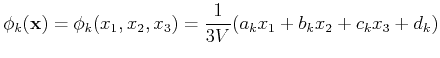(2.36)

where the coefficients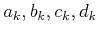are functions of the vertex coordinates and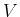is the volume of the element. Next, the following integral has to be calculated: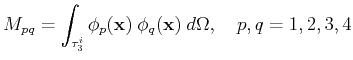(2.37)

The key for an efficient practical realization is that global finite elements are defined as transformations of a reference element in a normalized coordinate system. Each point of the cell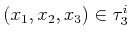can be expressed as a bijective function onto a reference point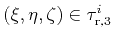: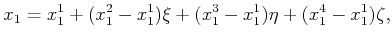(2.38)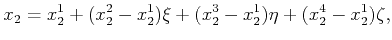(2.39)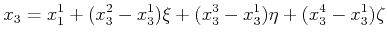(2.40)

The nodal basis functions on the reference element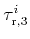are: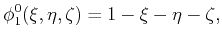(2.41)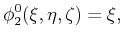(2.42)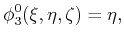(2.43)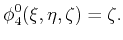(2.44)

Equation 2.37 is then calculated by: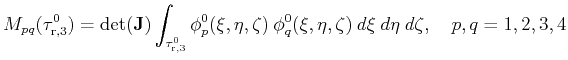(2.45)

where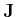is the Jacobian of the projection Equation 2.38 - Equation 2.40. The final assembly process consists of calculating stencil matrices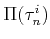for each element of the cell complex, mapping the local vertex indices to a global index and then building the global matrix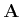.

For an electrostatic problem formulated by the Laplace equation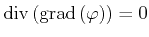the stencil matrix is represented by: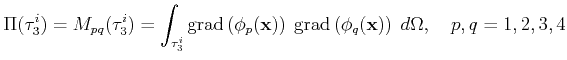(2.46)

This problem is linear and the global matrixis simply obtained by assembling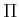with the corresponding index transformation.Next: 2.4 Finite Differences Up: 2. Numerical Discretization Schemes Previous: 2.2 Finite Volumes

R. Heinzl: Concepts for Scientific Computing